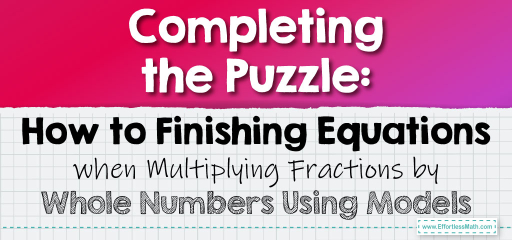# Completing the Puzzle: How to Finishing Equations when Multiplying Fractions by Whole Numbers Using Models

Visual models provide a tangible way to understand the multiplication of fractions by whole numbers.When presented with incomplete equations, these models can be instrumental in determining the missing value. In this guide, we’ll explore how to use visual models to finish equations that involve multiplying fractions by whole numbers.

## Step-by-step Guide to Finishing Equations when Multiplying Fractions by Whole Numbers Using Models:

1. Identifying the Model:

First, determine which visual model is being used in the equation. This could be an array, a number line, an area model, or a set model.

2. Interpreting the Model:

Examine the model to understand the given information:

– For arrays, count the shaded parts.

– For number lines, identify the starting and ending points.

– For area models, measure the shaded area.

– For set models, count the divided parts.

3. Completing the Equation:

Using the information from the model:

– Multiply the fraction by the whole number.

– Place the product in the missing part of the equation.

4. Simplifying the Result:

If the result is an improper fraction, convert it to a mixed number for a clearer representation.

### Example 1: (Array Model):

Given an array with 4 rows, each divided into 5 parts, and 3 parts shaded in each row, complete the equation: $$\_\_\_\_ \times \frac{3}{5} = \_\_\_\_$$

Solution:

There are 4 rows, so the whole number is 4.

Multiplying: $$4 \times \frac{3}{5} = \frac{12}{5} = 2 \frac{2}{5}$$

Completed equation: $$4 \times \frac{3}{5} = 2 \frac{2}{5}$$

The Absolute Best Book for 5th Grade Students

### 2. Example 2 (Number Line):

Given a number line starting at 0 and ending at 1.5 with jumps of $$\frac{1}{2}$$, complete the equation: $$3 \times \_\_\_\_ = 1.5$$

Solution:

There are 3 jumps of size $$\frac{1}{2}$$.

Completed equation: $$3 \times \frac{1}{2} = 1.5$$

### Practice Questions:

1. Given an area model of a rectangle with a length of 6 and a width divided into 4 parts, with 3 parts shaded, complete the equation: $$6 \times \_\_\_\_ = \_\_\_\_$$

2. Using a set model with 5 groups, each divided into 6 parts, and 4 parts taken from each group, complete the equation: $$5 \times \_\_\_\_ = \_\_\_\_$$

3. Given a number line starting at 0 and ending at 2.25 with jumps of $$\frac{3}{4}$$, complete the equation: $$\_\_\_\_ \times \frac{3}{4} = 2.25$$

A Perfect Book for Grade 5 Math Word Problems!

1. $$6 \times \frac{3}{4} = 4.5$$

2. $$5 \times \frac{4}{6} = 3 \frac{1}{3}$$

3. $$3 \times \frac{3}{4} = 2.25$$

The Best Math Books for Elementary Students

### What people say about "Completing the Puzzle: How to Finishing Equations when Multiplying Fractions by Whole Numbers Using Models - Effortless Math: We Help Students Learn to LOVE Mathematics"?

No one replied yet.

X
51% OFF

Limited time only!

Save Over 51%

SAVE $15 It was$29.99 now it is \$14.99